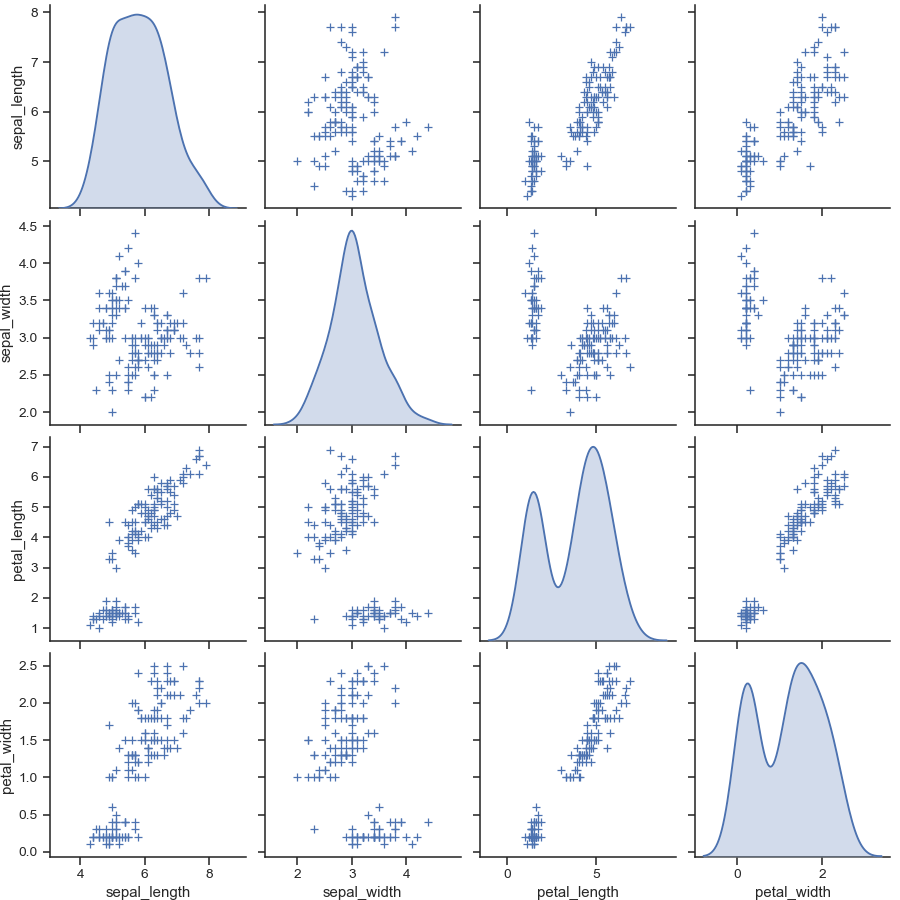# seaborn.pairplot¶

`seaborn.``pairplot`(data, hue=None, hue_order=None, palette=None, vars=None, x_vars=None, y_vars=None, kind='scatter', diag_kind='auto', markers=None, height=2.5, aspect=1, corner=False, dropna=True, plot_kws=None, diag_kws=None, grid_kws=None, size=None)

Plot pairwise relationships in a dataset.

By default, this function will create a grid of Axes such that each numeric variable in `data` will by shared in the y-axis across a single row and in the x-axis across a single column. The diagonal Axes are treated differently, drawing a plot to show the univariate distribution of the data for the variable in that column.

It is also possible to show a subset of variables or plot different variables on the rows and columns.

This is a high-level interface for `PairGrid` that is intended to make it easy to draw a few common styles. You should use `PairGrid` directly if you need more flexibility.

Parameters

Tidy (long-form) dataframe where each column is a variable and each row is an observation.

huestring (variable name), optional

Variable in `data` to map plot aspects to different colors.

hue_orderlist of strings

Order for the levels of the hue variable in the palette

palettedict or seaborn color palette

Set of colors for mapping the `hue` variable. If a dict, keys should be values in the `hue` variable.

varslist of variable names, optional

Variables within `data` to use, otherwise use every column with a numeric datatype.

{x, y}_varslists of variable names, optional

Variables within `data` to use separately for the rows and columns of the figure; i.e. to make a non-square plot.

kind{‘scatter’, ‘reg’}, optional

Kind of plot for the non-identity relationships.

diag_kind{‘auto’, ‘hist’, ‘kde’, None}, optional

Kind of plot for the diagonal subplots. The default depends on whether `"hue"` is used or not.

markerssingle matplotlib marker code or list, optional

Either the marker to use for all datapoints or a list of markers with a length the same as the number of levels in the hue variable so that differently colored points will also have different scatterplot markers.

heightscalar, optional

Height (in inches) of each facet.

aspectscalar, optional

Aspect * height gives the width (in inches) of each facet.

cornerbool, optional

If True, don’t add axes to the upper (off-diagonal) triangle of the grid, making this a “corner” plot.

dropnaboolean, optional

Drop missing values from the data before plotting.

{plot, diag, grid}_kwsdicts, optional

Dictionaries of keyword arguments. `plot_kws` are passed to the bivariate plotting function, `diag_kws` are passed to the univariate plotting function, and `grid_kws` are passed to the `PairGrid` constructor.

Returns
grid`PairGrid`

Returns the underlying `PairGrid` instance for further tweaking.

`PairGrid`

Subplot grid for more flexible plotting of pairwise relationships.

Examples

Draw scatterplots for joint relationships and histograms for univariate distributions:

```>>> import seaborn as sns; sns.set(style="ticks", color_codes=True)
>>> g = sns.pairplot(iris)
```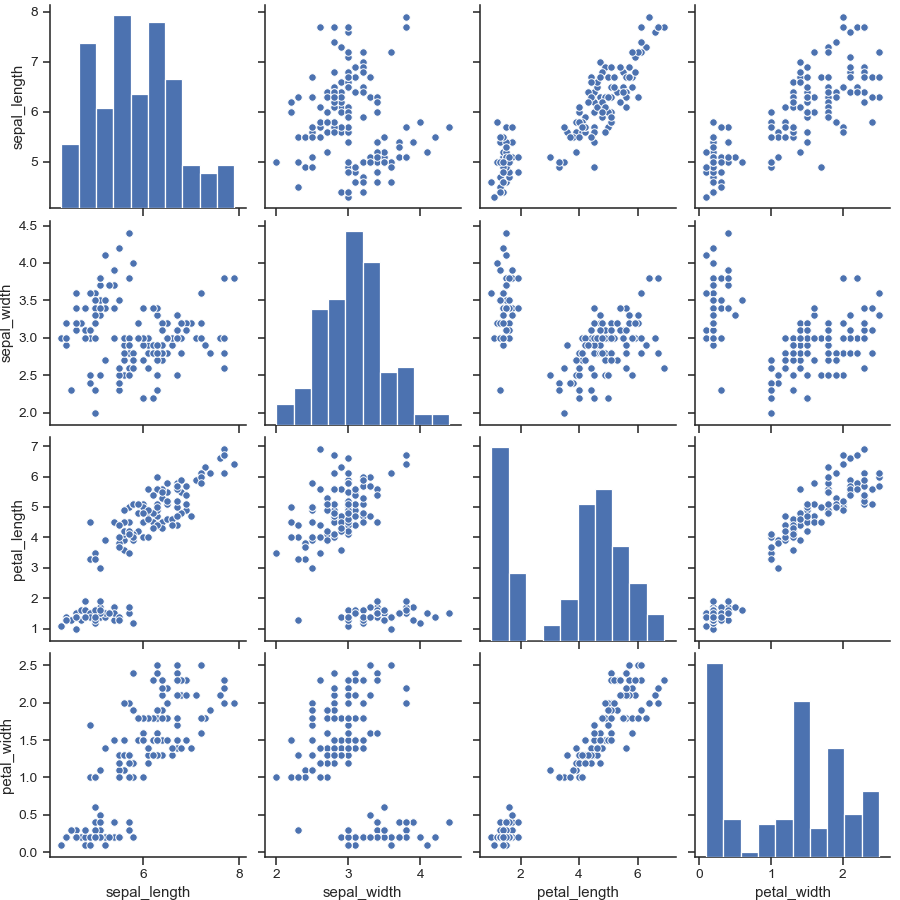Show different levels of a categorical variable by the color of plot elements:

```>>> g = sns.pairplot(iris, hue="species")
```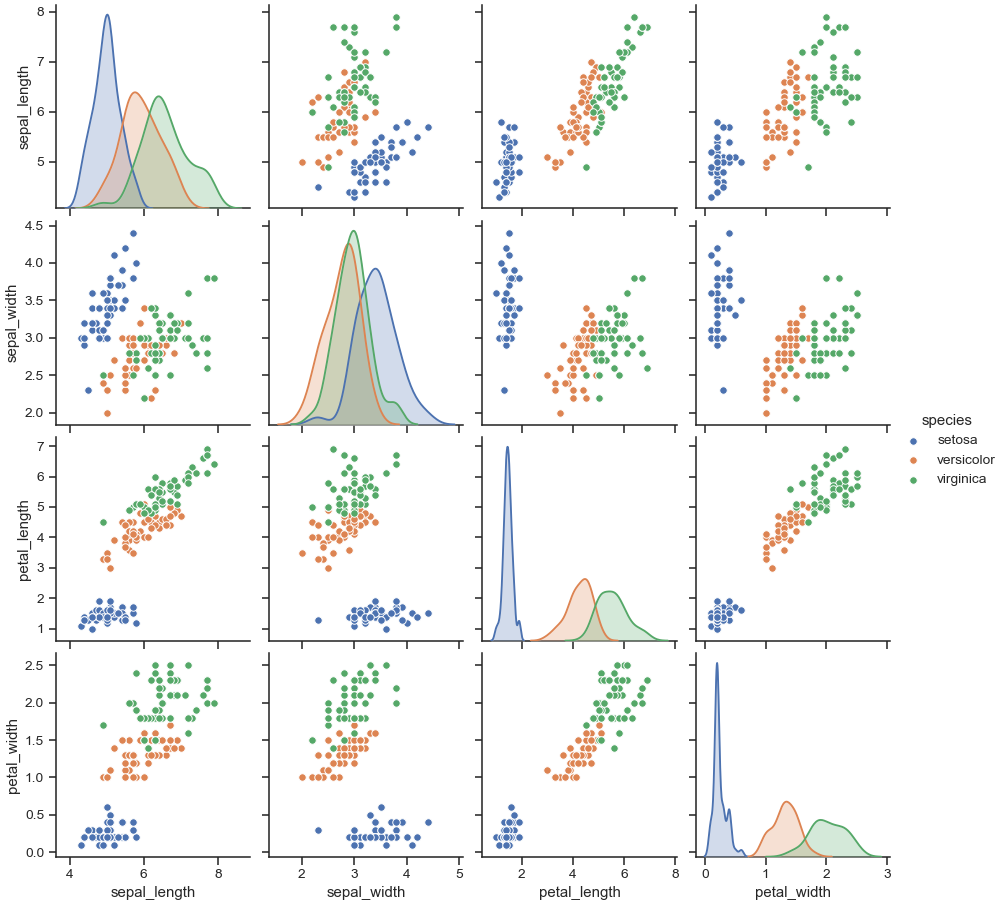Use a different color palette:

```>>> g = sns.pairplot(iris, hue="species", palette="husl")
```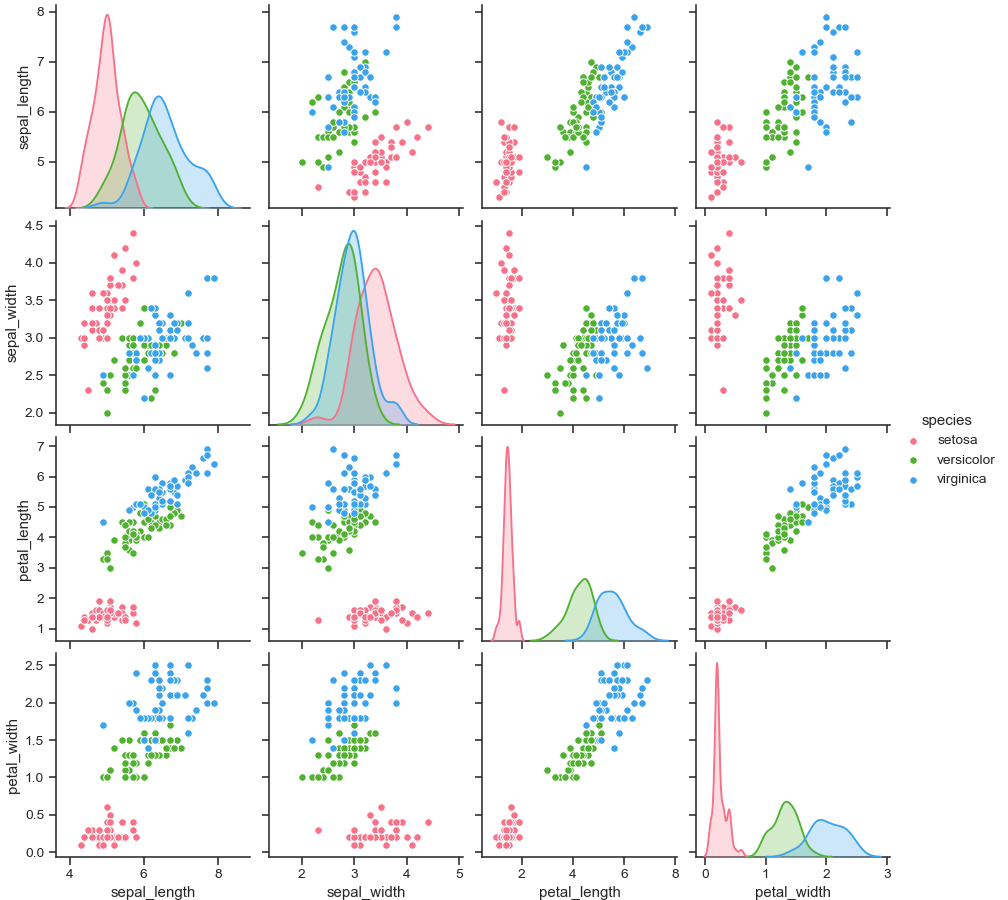Use different markers for each level of the hue variable:

```>>> g = sns.pairplot(iris, hue="species", markers=["o", "s", "D"])
```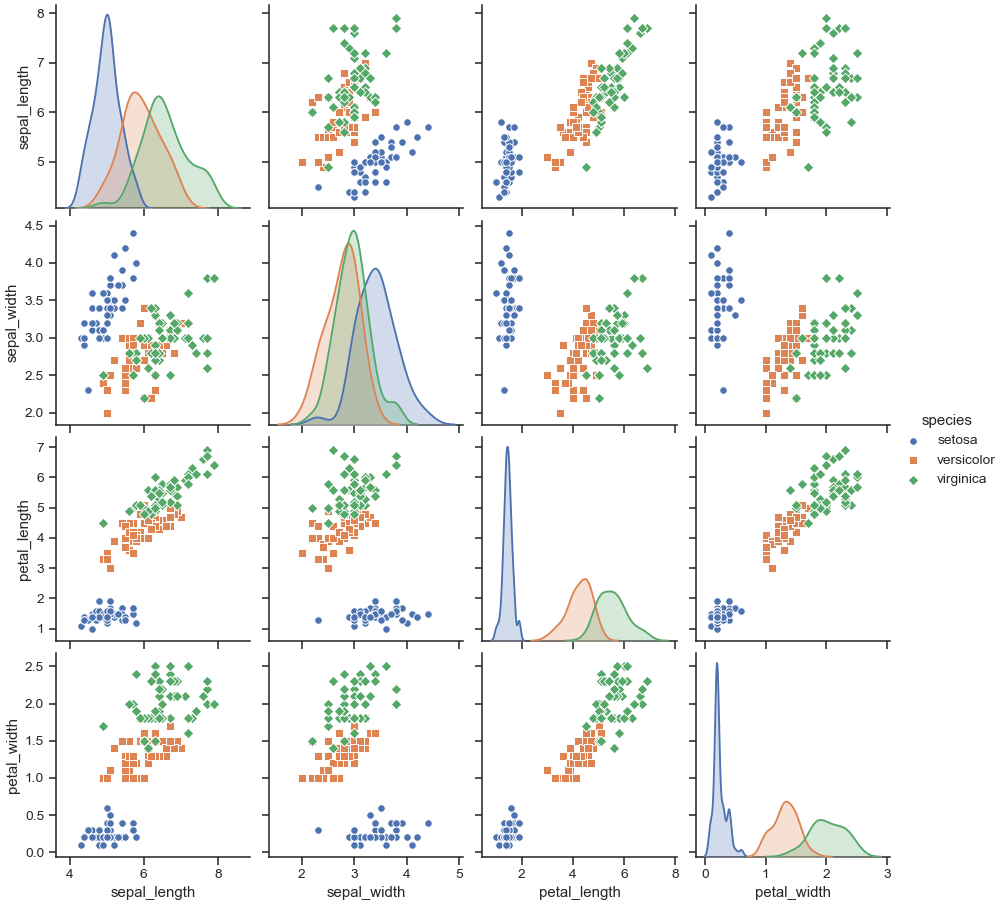Plot a subset of variables:

```>>> g = sns.pairplot(iris, vars=["sepal_width", "sepal_length"])
```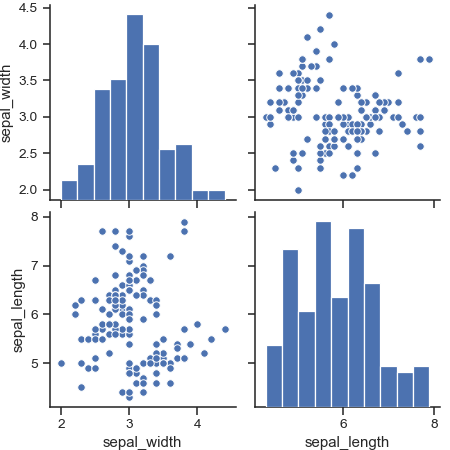Draw larger plots:

```>>> g = sns.pairplot(iris, height=3,
...                  vars=["sepal_width", "sepal_length"])
```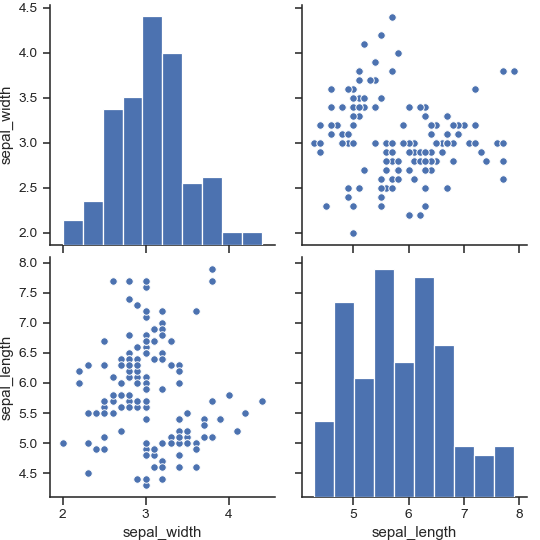Plot different variables in the rows and columns:

```>>> g = sns.pairplot(iris,
...                  x_vars=["sepal_width", "sepal_length"],
...                  y_vars=["petal_width", "petal_length"])
```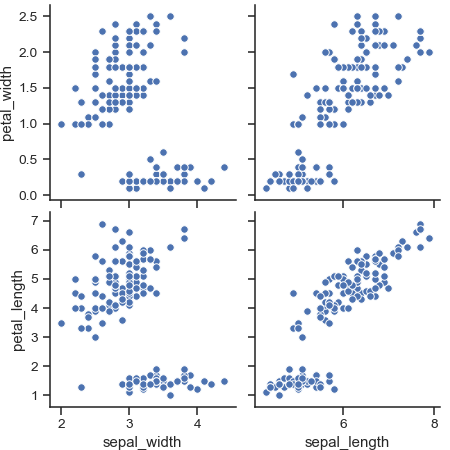Plot only the lower triangle of bivariate axes:

```>>> g = sns.pairplot(iris, corner=True)
```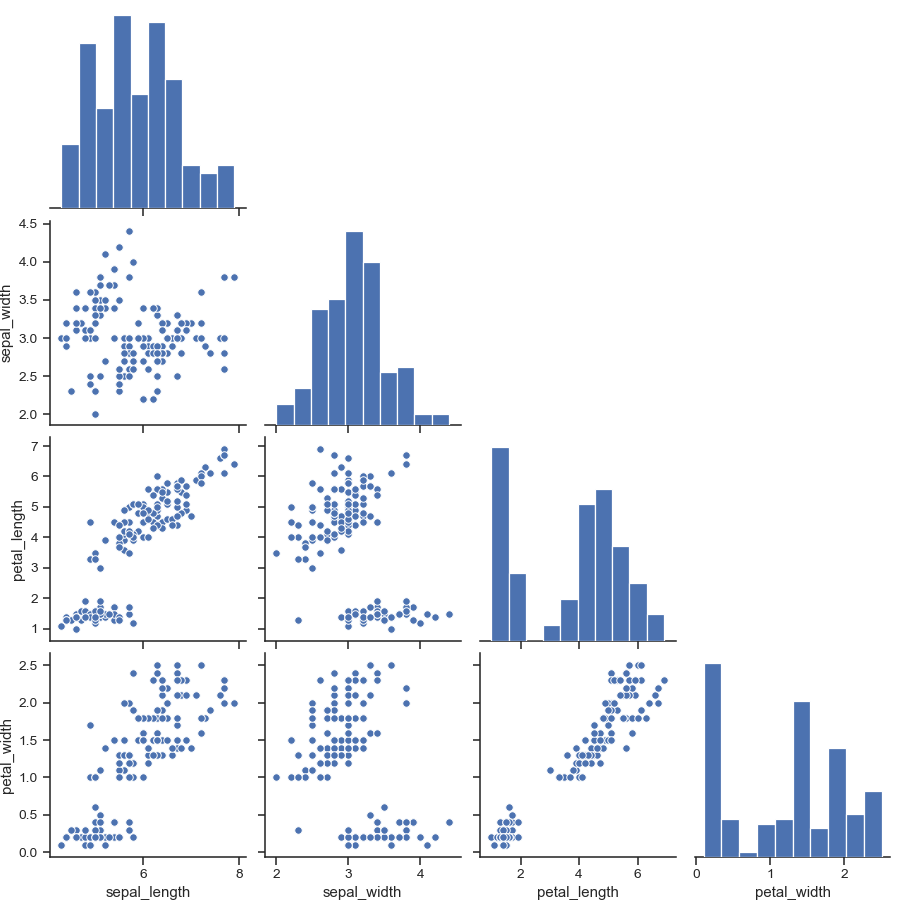Use kernel density estimates for univariate plots:

```>>> g = sns.pairplot(iris, diag_kind="kde")
```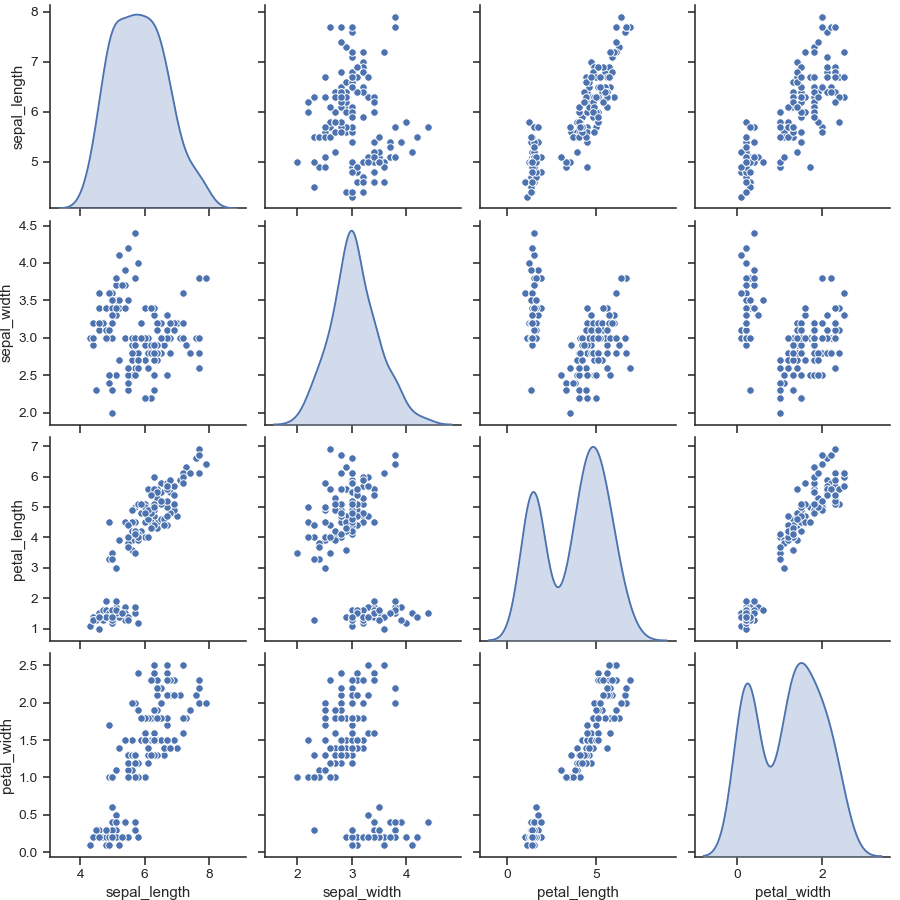Fit linear regression models to the scatter plots:

```>>> g = sns.pairplot(iris, kind="reg")
```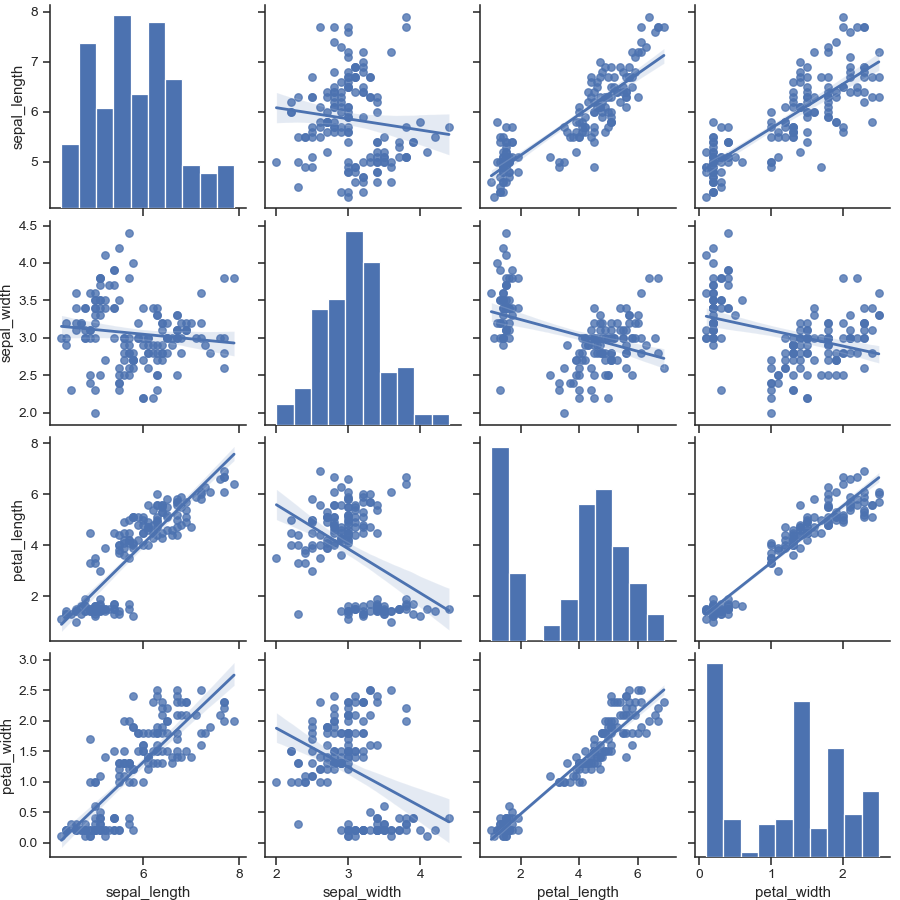Pass keyword arguments down to the underlying functions (it may be easier to use `PairGrid` directly):

```>>> g = sns.pairplot(iris, diag_kind="kde", markers="+",
...                  plot_kws=dict(s=50, edgecolor="b", linewidth=1),